Home | | Physics 11th std | Propagation of Sound Waves

# Propagation of Sound Waves

In the following section, we compute the speed of sound in air by Newton’s method and also discuss the Laplace correction and the factors affecting sound in air.

PROPAGATION OF SOUND WAVES

We know that sound waves are longitudinal waves, and when they propagate compressions and rarefactions are formed. In the following section, we compute the speed of sound in air by Newton’s method and also discuss the Laplace correction and the factors affecting sound in air.

## Newton’s formula for speed of sound waves in air

Sir Isaac Newton assumed that when sound propagates in air, the formation of compression and rarefaction takes place in a very slow manner so that the process is isothermal in nature. That is, the heat produced during compression (pressure increases, volume decreases), and heat lost during rarefaction (pressure decreases, volume increases) occur over a period of time such that the temperature of the medium remains constant. Therefore, by treating the air molecules to form an ideal gas, the changes in pressure and volume obey Boyle’s law, MathematicallyDifferentiating equation (11.20), we getwhere, BT is an isothermal bulk modulus of air. Substituting equation (11.21) in equation (11.16), the speed of sound in air is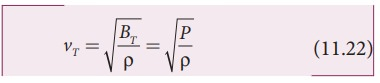Since P is the pressure of air whose value at NTP (Normal Temperature and Pressure) is 76 cm of mercury, we haveP = (0.76 × 13.6 ×103 × 9.8) N m-2

ρ= 1.293 kg m-3. here ρ is density of air

Then the speed of sound in air at Normal Temperature and Pressure (NTP) is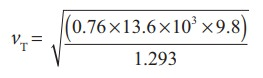=279.80 m s-1 ≈ 280 ms-1 (theoretical value)

But the speed of sound in air at 0°C is experimentally observed as 332 m s-1 which is close upto 16% more than theoretical value (Percentage error is ([332-280]/332 x 100% = 15.6%).

This error is not small

## Laplace’s correction

In 1816, Laplace satisfactorily corrected this discrepancy by assuming that when the sound propagates through a medium, the particles oscillate very rapidly such that the compression and rarefaction occur very fast. Hence the exchange of heat produced due to compression and cooling effect due to rarefaction do not take place, because, air (medium) is a bad conductor of heat. Since, temperature is no longer considered as a constant here, sound propagation is an adiabatic process. By adiabatic considerations, the gas obeys Poisson’s law (not Boyle’s law as Newton assumed), which is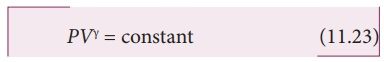which is the ratio between specific heat at constant pressure and specific heat at constant volume.

Differentiating equation (11.23) on both the sides, we getwhere, BA is the adiabatic bulk modulus of air. Now, substituting equation (11.24) in equation (11.16), the speed of sound in air isSince air contains mainly, nitrogen, oxygen, hydrogen etc, (diatomic gas), we take γ= 1.47. Hence, speed of sound in air is vA = ( √1.4)(280 m s-1)= 331.30 m s-1, which is very much closer to experimental data.

## Factors affecting speed of sound in gases

Let us consider an ideal gas whose equation of state iswhere, P is pressure, V is volume, T is temperature, n is number of mole and R is universal gas constant. For a given mass of a molecule, equation (11.26) can be written asFor a fixed mass m, density of the gas inversely varies with volume. i.e.,Substituting equation (11.28) in equation (11.27), we getwhere c is constant.

The speed of sound in air given in equation (11.25) can be written asFrom the above relation we observe the following

### (a) Effect of pressure :

For a fixed temperature, when the pressure varies, correspondingly density also varies such that the ratio (P/ρ) becomes constant.

This means that the speed of sound is independent of pressure for a fixed temperature. If the temperature remains same at the top and the bottom of a mountain then the speed of sound will remain same at these two points. But, in practice, the temperatures are not same at top and bottom of a mountain; hence, the speed of sound is different at different points.

### (b) Effect of temperature :the speed of sound varies directly to the square root of temperature in kelvin.

Let v0 be the speed of sound at temperature at 0° C or 273 K and v be the speed of sound at any arbitrary temperature T (in kelvin), thenSince v0 = 331m s-1 at 00C, v at any temperature in t0C is

v = (331 + 0.60t) m s-1

Thus the speed of sound in air increases by 0.61 m s-1 per degree celcius rise in temperature. Note that when the temperature is increased, the molecules will vibrate faster due to gain in thermal energy and hence, speed of sound increases.

### (c) Effect of density :

Let us consider two gases with different densities having same temperature and pressure. Then the speed of sound in the two gases are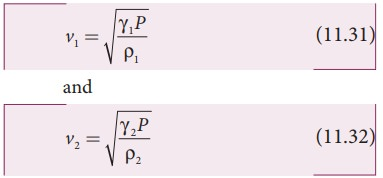Taking ratio of equation (11.31) and equation (11.32), we get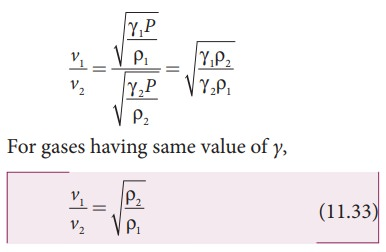Thus the velocity of sound in a gas is inversely proportional to the square root of the density of the gas.

### (d) Effect of moisture (humidity):

We know that density of moist air is 0.625 of that of dry air, which means the presence of moisture in air (increase in humidity) decreases its density. Therefore, speed of sound increases with rise in humidity. From equation (11.30)Let ρ1, v1 and ρ2, v2 be the density and speeds of sound in dry air and moist air, respectively. Then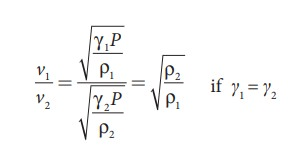Since P is the total atmospheric pressure, it can be shown thatwhere p1 and p2 are the partial pressures of dry air and water vapour respectively. Then### (e) Effect of wind:

The speed of sound is also affected by blowing of wind. In the direction along the wind blowing, the speed of sound increases whereas in the direction opposite to wind blowing, the speed of sound decreases.

### EXAMPLE 11.9

The ratio of the densities of oxygen and nitrogen is 16:14. Calculate the temperature when the speed of sound in nitrogen gas at 17°C is equal to the speed of sound in oxygen gas.

### Solution

From equation (11.25), we haveWhere, R is the universal gas constant and M is the molecular mass of the gas. The speed of sound in nitrogen gas at 17°C isSimilarly, the speed of sound in oxygen gas at t in K isGiven that the value of γ is same for both the gases, the two speeds must be equal. Hence, equating equation (1) and (2), we getSquaring on both sides and cancelling γ R term and rearranging, we getSince the densities of oxygen and nitrogen is 16:14,Substituting equation (5) in equation (3), we getStudy Material, Lecturing Notes, Assignment, Reference, Wiki description explanation, brief detail
11th Physics : UNIT 11 : Waves : Propagation of Sound Waves |